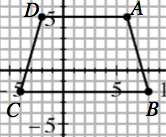### Home > CC1 > Chapter 5 > Lesson 5.3.3 > Problem5-93

5-93.

Graph the trapezoid $A(6, 5), B(8, −2), C(−4, −2), D(−2, 5)$.

Your graph should look like the one below.1. Find the length of the bottom base (segment $CB$). Then find the length of the top base (segment $AD$). Use grid units.

• Both $B$ and $C$ are on the $y = −2$ line, so you just need to find the the distance between their x-coordinates to determine the length of CB. The same can be done for AD.

$\text{The length of segment }CB \text{ is }\left | 8 \right| + \left | -4 \right | =\ 12.\text{ Now find the length of segment } AD.$

1. Find the distance between the two bases, which is called the height. Use grid units.

• Use a similar method to part (a), but use the y-coordinates to find the distance between segment $CB$ and segment $AD$.